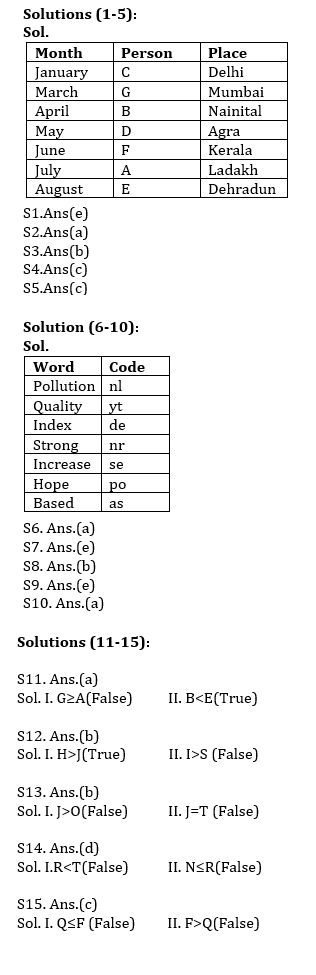Latest Banking jobs   »   Reasoning Ability Quiz For IBPS RRB...

# Reasoning Ability Quiz For IBPS RRB PO, Clerk Prelims 2021- 19th June

Directions (1-5): Study the information carefully and answer the questions given below.
Seven persons A, B, C, D, E, F and G are going to vacation in six different months i.e. January, March, April, May, June, July and August to six different places i.e. Dehradun, Delhi, Mumbai, Agra, Ladakh, Nainital and Kerala. All information is not necessarily in same order.
B goes in the month which has even number of days. Two persons goes between B and the one who goes Ladakh. The one who goes Agra go just before the one who goes Kerala. The one who goes Kerala go in the month which has even number of days. Only one person goes between the one who goes Agra and G, who goes Mumbai. D goes after B. Four persons goes between A and the one who goes Delhi. The one who goes Dehradun goes after the one who goes Delhi. B does not go Dehradun. Neither D nor C goes Kerala and Dehradun. F goes just after D.

Q1.Who among the following person goes Dehradun?
(a) A
(b) B
(c) F
(d) The one who goes just after B
(e) None of these

Q2.Who among the following person goes in June?
(a) The one who goes Kerala
(b) B
(c) The one who goes Ladakh
(d) E
(e) None of these

Q3.Who among the following person goes just before B?
(a) D
(b) G
(c) B
(d) F
(e) None of these

Q4. Which of the following combination is true?
(a) G-Nainital
(b) C-Mumbai
(c) D-Agra
(d) A-kerala
(e) None is true

Q5. Four of the following five are alike in certain way based from a group, find the one that does not belong to that group?
(a) C
(b) D
(c) B
(d) E
(e) G

Directions (6-10): Study the following information carefully and answer the questions given below:
In a certain code language,
‘Pollution Quality Index’ is written as ‘nl yt de’,
‘Strong increase hope’ is written as ‘po se nr’,
‘Quality Based Strong’ is written as ‘yt as nr’
‘Index Strong Hope’ is written as ‘nr de po’.

Q6. What is the code for ‘Pollution increase’ in the given code language?
(a) nl se
(b) nl po
(c) nr se
(d) de se
(e) None of these

Q7. What is the code for ‘Based’ in the given code language?
(a) po
(b) se
(c) nr
(d) yt
(e) None of these

Q8. What may be the code for ‘Strong opinion’ in the given code language?
(a) nr po
(b) nr fg
(c) se po
(d) nl de
(e) nr as

Q9. What is the code for ‘increase’ in the given code language?
(a) as
(b) nr
(c) po
(d) nl
(e) None of these

Q10. What may be the code for ‘Quality assured Index’?
(a) yt de dg
(b) nl yt as
(c) nr as yt
(d) de dg po
(e) po yt de

Directions (11-15): In these questions, a relationship between different elements is shown in the statements. The statements are followed by two conclusions. Give answer

Q11. Statement: A>B; G<D≤E; G≥F>B
Conclusion: I. G≥A II. B<E
(a) if only conclusion II is true.
(b) if only conclusion I is true.
(c) if neither conclusion I nor II is true.
(d) if either conclusion I or II is true.
(e) if both conclusions I and II are true.

Q12. Statement: A>T≥J; A≤S=H; I>T
Conclusion: I. H>J II. I>S
(a) if both conclusion I and II are true.
(b) if only conclusion I is true.
(c) if neither conclusion I nor II is true.
(d) if either conclusion I or II is true.
(e) if only conclusion II is true.

Q13. Statement: J≥K>L<M≥N; K≥O=T
Conclusion: I. J>O II. J=T
(a) if only conclusion II is true.
(b) if either conclusion I or II is true.
(c) if neither conclusion I nor II is true.
(d) if only conclusion I is true.
(e) if both conclusions I and II are true.

Q14. Statement: A> T=N; A>S>R; A<M
Conclusion: I. R<T II. N≤R
(a) if only conclusion II is true.
(b) if only conclusion I is true.
(c) if neither conclusion I nor II is true.
(d) if either conclusion I or II is true.
(e) if both conclusions I and II are true.

Q15. Statement: A<B≤C; F<M≤C; C>Q
Conclusion: I. Q≤F II. F>Q
(a) if only conclusion II is true.
(b) if either conclusion I or II is true.
(c) if neither conclusion I nor II is true.
(d) if only conclusion I is true.
(e) if both conclusions I and II are true.

Practice More Questions of Reasoning for Competitive Exams:

###### Study Plan for IBPS RRB PO/Clerk Prelims 2021

Solutions